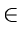Next:10.3 Examples of some Intractable ProblemsUp:10. Introduction to NP-CompletenessPrevious:10.1 Importance of NP-Completeness

# 10.2 Optimization Problems and Decision Problems

NP-completeness has been studied in the framework of decision problems. Most problems are not decision problems, but optimization problems (where some value needs to be minimized or maximized). In order to apply the theory of NP-completeness to optimization problems, we must recast them as decision problems. We provide an example of how an optimization problem can be transformed into a decision problem.

Example: Consider the problem SHORTEST-PATH that finds a shortest path between two given vertices in an unweighted, undirected graph G = (V, E). An instance of SHORTEST-PATH consists of a particular graph and two vertices of that graph. A solution is a sequence of vertices in the graph, with perhaps an empty sequence denoting that no path exists. Thus the problem SHORTEST-PATH is a relation that associates each instance of a graph and two vertices with a solution (namely a shortest path in this case). Note that a given instance may have no solution, exactly one solution, or multiple solutions.

A decision problem PATH related to the SHORTEST-PATH problem above is : Given a graph G = (V, E), two vertices u, vV, and a non-negative integer k, does a path exist in G between u and v whose length is at most k ?

Note that the decision problem PATH is one way of casting the original optimization problem as a decision problem. We have done this by imposing a bound on the value to be optimized. This is a popular way of transforming an optimization problem into a decision problem.

If an optimization problem is easy then its related decision problem is easy as well. Similarly, if we can provide evidence that a decision problem is hard, we also provide evidence that its related optimization problem is hard.Next:10.3 Examples of some Intractable ProblemsUp:10. Introduction to NP-CompletenessPrevious:10.1 Importance of NP-Completeness
eEL,CSA_Dept,IISc,Bangalore